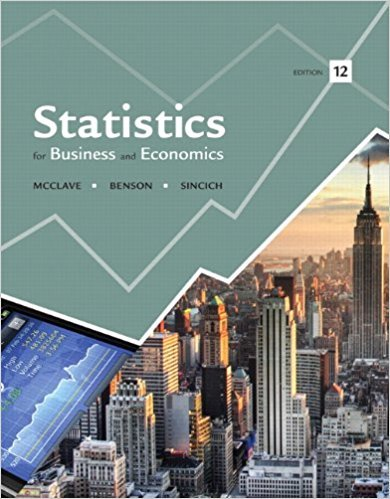×
×

# Use of country club facilities. A local country club has aISBN: 9780321826237 51

## Solution for problem 107SE Chapter 3

Statistics for Business and Economics | 12th Edition

• Textbook Solutions
• 2901 Step-by-step solutions solved by professors and subject experts
• Get 24/7 help from StudySoup virtual teaching assistantsStatistics for Business and Economics | 12th Edition

4 5 1 287 Reviews
11
0
Problem 107SE

Use of country club facilities. A local country club has a membership of 600 and operates facilities that include an 18-hole championship golf course and 12 tennis courts. Before deciding whether to accept new members, the club president would like to know how many members regularly use each facility. A survey of the membership indicates that 70% regularly use the golf course, 50% regularly use the tennis courts, and 5% use neither of these facilities regularly.

a. Construct a Venn diagram to describe the results of the survey.

b. If one club member is chosen at random, what is the probability that the member uses either the golf course or the tennis courts or both?

c. If one member is chosen at random, what is the probability that the member uses both the golf and the tennis facilities?

d. A member is chosen at random from among those known to use the tennis courts regularly. What is the probability that the member also uses the golf course regularly?

Step-by-Step Solution:

Step 1 of 5:

It is given that a local country club has a membership of 600 operates facilities.

It includes 10-hole championship golf course and 12 tennis courts.

A survey indicated that 70% of the members regularly use the golf course, 50% of the members regularly use tennis courts and 5% of the members use neither of the two regularly.

Using this data we need to find the required answers.

Step 2 of 5:

(a)

Here we need to construct a Venn diagram to represent the data.

Let us define the events as

A=members regularly using golf course

B=members regularly using tennis court

C=members regularly using neither facilities

We have

P(A)=0.70

P(B)=0.50

P(C)=0.05.

Now the probability that a member uses at least one of the facility is

P()=1-P(C)

=1-0.05

=0.95

The probability that a member uses both the facilities is denoted by P(AB). It can be calculated using additive property.

We have P()=P(AB).

P(AB)=P(A)+P(B)-P(AB)

0.95=0.70+0.50-P(AB)P(AB)=1.2-0.95

=0.25

Thus, the Venn diagram becomesStep 3 of 5:

(b)

Here we need to find the probability that a randomly chosen member uses either any one of the facility or both the facilities.

It is given by P(AB).

We have P(AB)=0.95

Thus, the probability that a randomly chosen member will use either one of the facility of both the facilities is 0.95.

Step 4 of 5

Step 5 of 5

##### ISBN: 9780321826237

This textbook survival guide was created for the textbook: Statistics for Business and Economics , edition: 12. This full solution covers the following key subjects: regularly, member, use, Golf, Tennis. This expansive textbook survival guide covers 15 chapters, and 1630 solutions. The full step-by-step solution to problem: 107SE from chapter: 3 was answered by , our top Business solution expert on 07/21/17, 05:42AM. Statistics for Business and Economics was written by and is associated to the ISBN: 9780321826237. Since the solution to 107SE from 3 chapter was answered, more than 2289 students have viewed the full step-by-step answer. The answer to “Use of country club facilities. A local country club has a membership of 600 and operates facilities that include an 18-hole championship golf course and 12 tennis courts. Before deciding whether to accept new members, the club president would like to know how many members regularly use each facility. A survey of the membership indicates that 70% regularly use the golf course, 50% regularly use the tennis courts, and 5% use neither of these facilities regularly.a. Construct a Venn diagram to describe the results of the survey.b. If one club member is chosen at random, what is the probability that the member uses either the golf course or the tennis courts or both?c. If one member is chosen at random, what is the probability that the member uses both the golf and the tennis facilities?d. A member is chosen at random from among those known to use the tennis courts regularly. What is the probability that the member also uses the golf course regularly?” is broken down into a number of easy to follow steps, and 164 words.

Unlock Textbook Solution